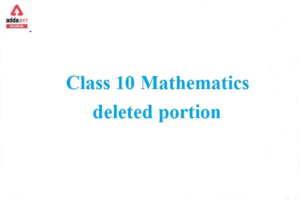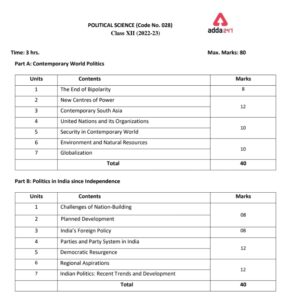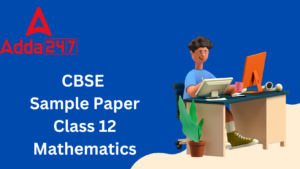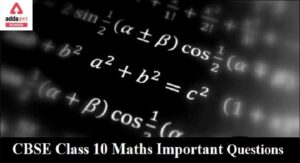Online Tution   »   CBSE Syllabus - Class 9,10, 11,...   »   CBSE Class 10 Maths Syllabus 2022-23

## CBSE Class 10 Maths Syllabus 2022-23

Students can get the CBSE syllabus for classes 10 and 12 on the official website. The CBSE class 10 mathematics syllabus 2023 will help the students to cover all the topics without leaving any topics behind. Mathematics is always considered a difficult subject but if students go through step by step process it will be easier for them to understand the sums. Mathematics is an important subject as it will be a core subject in higher studies. In this article, we have covered CBSE class 10 mathematics syllabus 2023 including topic-wise marks distribution, unit-wise summary, and question paper pattern. Students should always prefer the CBSE class 10 syllabus 2023 to prepare for the exam.

## Class 10 Maths Syllabus 2022-23

CBSE class 10 maths syllabus 2023 consists of six units, number system, Algebra, coordinate geometry, geometry, mensuration, and statistics and probability. All the units with weightage for the class 10 board exams are listed below. Students can go through the units and understand the marking scheme for each unit so that they can know which unit they need to focus on.

 Sl no. UNIT MARKS 1. Number system 10 2. Algebra 20 3. Coordinate Geometry 04 4. Geometry 27 5. Mensuration 13 6. Statistics & probability 06 Total 80 Internal Assessments 20 Total marks 100

## Maths Class 10 Syllabus 2022-23: Unit wise Summary

### CBSE Class 10 Maths Syllabus 2022-23:UNIT I- NUMBER SYSTEMS

1. REAL NUMBERS (18) Periods
• Review of representation of natural numbers, integers, and rational numbers on the number line. Rational numbers as recurring/ terminating decimals. Operations on real numbers.
• Examples of non-recurring/non-terminating decimals. Existence of non-rational numbers (irrational numbers) such as, and their representation on the number line. Explaining that every real number is represented by a unique point on the number line and conversely, viz. every point on the number line represents a unique real number.
• Definition of nth root of a real number.
• Rationalization (with precise meaning) of real numbers of type and  (and their combinations) where x and y are natural numbers and a and b are integers.
• Recall of laws of exponents with integral powers. Rational exponents with positive real bases (to be done by particular cases, allowing the learner to arrive at the general laws.)

Total number of periods- 18 periods

Total marks- 10

### CBSE Class 10 Maths Syllabus 2022-23 UNIT II: ALGEBRA

• POLYNOMIALS (26) Periods

Definition of a polynomial in one variable, with examples and counterexamples. Coefficients of a polynomial, terms of a polynomial, and zero polynomial. Degree of a polynomial. Constant, linear, quadratic, and cubic polynomials. Monomials, binomials, trinomials. Factors and multiples. Zeros of a polynomial. Motivate and State the Remainder Theorem with examples. Statement and proof of the Factor Theorem. Factorization of ax2+ bx + c, a ≠ 0 where a, b and c are real numbers, and of cubic polynomials using the Factor Theorem.

Recall algebraic expressions and identities. Verification of identities:

and their use in the factorization of polynomials.

• LINEAR EQUATIONS IN TWO VARIABLES (16) Periods

Recall linear equations in one variable. Introduction to the equation in two variables. Focus on linear equations of the type ax + by + c=0.Explain that a linear equation in two variables has infinitely many solutions and justify their being written as ordered pairs of real numbers, plotting them and showing that they lie on a line.

Total number of periods- 42

Total marks- 20

### CBSE Class 10 Maths Syllabus 2022-23 UNIT III: COORDINATE GEOMETRY

• COORDINATE GEOMETRY

The Cartesian plane, coordinates of a point, names and terms associated with the coordinate plane, notations.

Total number of periods- 7

Total marks- 04

### CBSE Class 10 Maths Syllabus 2022-23 UNIT IV: GEOMETRY

• INTRODUCTION TO EUCLID’S GEOMETRY      (7) Periods

History – Geometry in India and Euclid’s geometry. Euclid’s method of formalizing observed phenomena into rigorous mathematics with definitions, common/obvious notions, axioms/postulates, and theorems. The five postulates of Euclid. Showing the relationship between axiom and theorem, for example:

(Axiom) 1. Given two distinct points, there exists one and only one line through them.

(Theorem) 2. (Prove) Two distinct lines cannot have more than one point in common.

• LINES AND ANGLES    (15) Periods
1. (Motivate) If a ray stands on a line, then the sum of the two adjacent angles so formed is 180O and the converse.
2. (Prove) If two lines intersect, vertically opposite angles are equal.
3. (Motivate) Lines that are parallel to a given line are parallel.
• TRIANGLES (22) Periods
1. (Motivate) Two triangles are congruent if any two sides and the included angle of one triangle is equal to any two sides and the included angle of the other triangle (SAS Congruence).
2. (Prove) Two triangles are congruent if any two angles and the included side of one triangle is equal to any two angles and the included side of the other triangle (ASA Congruence).
3. (Motivate) Two triangles are congruent if the three sides of one triangle are equal to three sides of the other triangle (SSS Congruence).
4. (Motivate) Two right triangles are congruent if the hypotenuse and a side of one triangle are equal (respectively) to the hypotenuse and a side of the other triangle. (RHS Congruence)
5. (Prove) The angles opposite to equal sides of a triangle are equal.
6. (Motivate) The sides opposite to equal angles of a triangle are equal.
1. (Prove) The diagonal divides a parallelogram into two congruent triangles.
2. (Motivate) In a parallelogram opposite sides are equal, and conversely.
3. (Motivate) In a parallelogram opposite angles are equal, and conversely.
4. (Motivate) A quadrilateral is a parallelogram if a pair of its opposite sides are parallel and equal.
5. (Motivate) In a parallelogram, the diagonals bisect each other and conversely.
6. (Motivate) In a triangle, the line segment joining the midpoints of any two sides is parallel to the third side, and in half of it, and (motivate) its converse.
• CIRCLES    (17) Periods
1. (Prove) Equal chords of a circle subtend equal angles at the center and (motivate) its converse.
2. (Motivate) The perpendicular from the center of a circle to a chord bisects the chord and conversely, the line is drawn through the center of a circle to bisect a chord is perpendicular to the chord.
3. (Motivate) Equal chords of a circle (or of congruent circles) are equidistant from the center (or their respective centers) and conversely.
4. (Prove) The angle subtended by an arc at the center is double the angle subtended by it at any point on the remaining part of the circle.
5. (Motivate) Angles in the same segment of a circle are equal.
6. (Motivate) If a line segment joining two points subtends an equal angle at two other points lying on the same side of the line containing the segment, the four points lie on a circle.
7. (Motivate) The sum of either of the pair of the opposite angles of a cyclic quadrilateral is 180° and its converse.

Total numbers of periods- 74

Total Marks- 27

### CBSE Class 10 Maths Syllabus 2022-23 UNIT V: MENSURATION

• AREAS      (5) Periods

Area of a triangle using Heron’s formula (without proof)

• SURFACE AREAS AND VOLUMES      (17) Periods

Surface areas and volumes of spheres (including hemispheres) and right circular cones.

Total number of periods- 22

Total marks- 13

### CBSE Class 10 Maths Syllabus 2022-23 UNIT VI: STATISTICS & PROBABILITY

• STATISTICS                                                                                                                                 (15) Periods

Bar graphs, histograms (with varying base lengths), and frequency polygons.

Total number of periods- 15

Total marks- 06

## CBSE Class 10 Mathematics Syllabus 2022-23 Reference Books

1. Mathematics – Textbook for Class IX – NCERT Publication
2. Mathematics – Textbook for Class X – NCERT Publication
3. Guidelines for Mathematics Laboratory in Schools, class IX – CBSE Publication
4. Guidelines for Mathematics Laboratory in Schools, class X – CBSE Publication
5. Laboratory Manual – Mathematics, secondary stage – NCERT Publication
6. Mathematics exemplar problems for class IX, NCERT publication.
7. Mathematics exemplar problems for class X, NCERT publication

## CBSE Class 10 Maths syllabus 2022-23: Question Paper Design

Question paper design includes the types of questions expected, total marks of each section, and weightage in percentage. This year two types of question paper designs are provided by CBSE for the students, one is basic and the other is standard. Check out both the question paper design below to get a detailed view of the CBSE class 10 mathematics syllabus 2023.

External Assessment

1. Standard question paper design

 S. No. Typology of Questions Total Marks % Weightage (approx.) 1 ·        Remembering ·        Understanding 43 54 2 ·        Applying 19 24 3 ·        Analysing:   ·        Evaluating:   ·        Creating: 18 22 Total 80 100

1. Basic question paper design
 S. No. Typology of Questions Total Marks % Weightage (approx.) 1 ·        Remembering ·        Understanding 60 75 2 ·        Applying 12 15 3 ·        Analysing ·        Evaluating   ·        Creating 8 10 ·        Total 80 100

Internal Assessments

1. Standard Internal Question paper design
 INTERNAL ASSESSMENT MARKS Pen Paper Test and Multiple Assessment (5+5) 10 Marks Portfolio 05 Marks Lab Practical (Lab activities to be done from the prescribed books) 05 Marks
1. Basic Internal Question paper design
 INTERNAL ASSESSMENT MARKS Pen Paper Test and Multiple Assessment  (5+5) 10 Marks Portfolio 05 Marks Lab Practical (Lab activities to be done from the prescribed books) 05 Marks

## CBSE Class 10 Mathematics Syllabus 2022-23: FAQs

1. What is the syllabus of maths for CBSE class 10?

Ans. To know the syllabus of CBSE class 10th mathematics syllabus 2022-2023 go to the official website. For the year 2023, the syllabus of maths for class 10th includes a number system, algebra, geometry, coordinate geometry, mensuration, and statistics and probability.

1. How to prepare for mathematics in the class 10 board exam?

Ans. Mathematics as a subject requires a lot of hard work, attention, and practice. To score higher in this subject students have to practice all the sums regularly. The student should be familiar with the CBSE class 10 syllabus 2023 and should study according to the syllabus to score good marks in the board exams.

1. Is mathematics a good scoring subject in class 10?

Ans. Yes, mathematics is a good scoring subject in class 10th the most weightage is given to geometry which is of 27 marks. Geometry relatively is an easier topic compared to the other units of mathematics. Students can prepare well by following the CBSE class 10th mathematics syllabus 2023.

1. What is the scope of maths in class 10?

Ans. If the student practices well according to the CBSE syllabus for class 10th 2023 then the scope of scoring good marks in maths is high. The student should practice and memorize all the formulas and clear their concepts in the subject of maths to score good marks. The CBSE class 10th mathematics syllabus 2023 includes units like number system, algebra, co-ordinate geometry, geometry, mensuration, and statistics and probability

Sharing is caring!

## FAQs

### What is the syllabus of maths for CBSE class 10?

To know the syllabus of CBSE class 10th mathematics syllabus 2022-2023 go to the official website. For the year 2023, the syllabus of maths for class 10th includes a number system, algebra, geometry, coordinate geometry, mensuration, and statistics and probability.

### How to prepare for mathematics in the class 10 board exam?

Mathematics as a subject requires a lot of hard work, attention, and practice. To score higher in this subject students have to practice all the sums regularly. The student should be familiar with the CBSE class 10 syllabus 2023 and should study according to the syllabus to score good marks in the board exams.

### Is mathematics a good scoring subject in class 10?

Yes, mathematics is a good scoring subject in class 10th the most weightage is given to geometry which is of 27 marks. Geometry relatively is an easier topic compared to the other units of mathematics. Students can prepare well by following the CBSE class 10th mathematics syllabus 2023.

### What is the scope of maths in class 10?

If the student practices well according to the CBSE syllabus for class 10th 2023 then the scope of scoring good marks in maths is high. The student should practice and memorize all the formulas and clear their concepts in the subject of maths to score good marks. The CBSE class 10th mathematics syllabus 2023 includes units like number system, algebra, co-ordinate geometry, geometry, mensuration, and statistics and probability

### TOPICS:

•Class 10 Maths Deleted Syllabus 2023-24,...
•CBSE Class 12 Informatics Practices Syll...
•Political Science Class 12 Syllabus 2022...
•Maths Sample Paper Class 12 2023 with CB...
•Previous Year Question Papers PYQ Class ...
•CBSE Important Questions for Class 10 Ma...